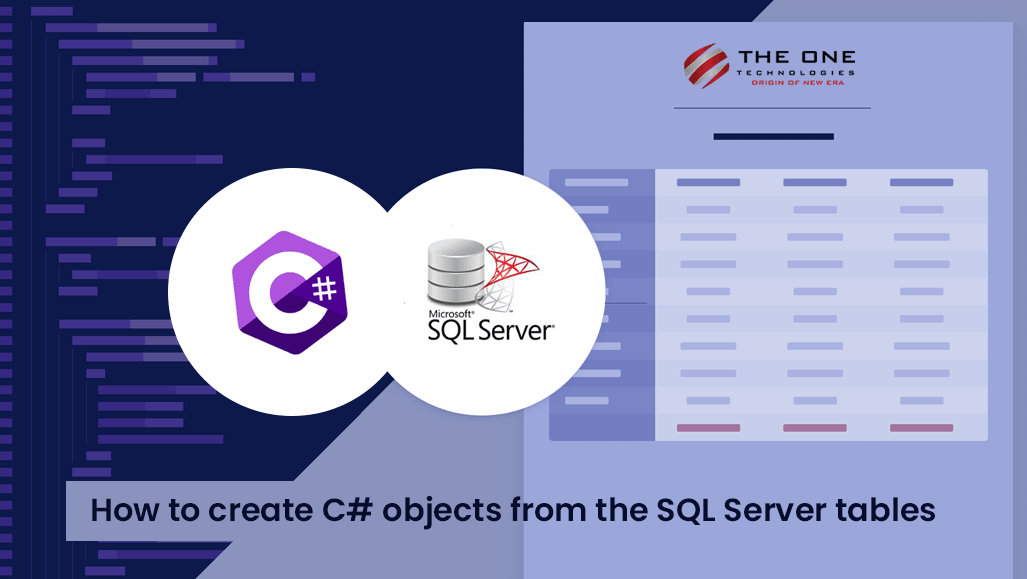# How to Create C# Objects From The SQL Server Tables## Introduction

We can come across a moment when we need the data of the tables as objects to be used in the code for multiple discrete uses.

## The use cases are as below:

1. We need these objects in database seeding, where we list the C# objects in the code and make it insert or update while the app starts.
``````/// <summary>
/// Intializes the Data
/// </summary>
/// <param name="services"></param>
{
using (var dbContext = services.GetService<ApplicationDbContext>())
{
if (!dbContext.TestTable.Any())
{
{
new TestTable() { Id = 1, Field1 = 1, Field2 = "Rossy", Field3 = 90.14 },
new TestTable() { Id = 2, Field1 = 27, Field2 = "Barnie", Field3 = 2.85 },
new TestTable() { Id = 3, Field1 = 48, Field2 = "Bunnie", Field3 = 10.16 },
new TestTable() { Id = 4, Field1 = 50, Field2 = "Rockwell", Field3 = 60.35 },
new TestTable() { Id = 5, Field1 = 89, Field2 = "Clarey", Field3 = 25.97 },
new TestTable() { Id = 6, Field1 = 63, Field2 = "Matt", Field3 = 68.73 },
new TestTable() { Id = 7, Field1 = 77, Field2 = "Amble", Field3 = 25.12 },
new TestTable() { Id = 8, Field1 = 95, Field2 = "Marti", Field3 = 77.49 },
new TestTable() { Id = 9, Field1 = 97, Field2 = "linnie", Field3 = 54.03 },
new TestTable() { Id = 10, Field1 = 99, Field2 = "Briano", Field3 = 27.33 }
});

await dbContext.SaveChangesAsync();
}
}
}``````
1. We need these objects in the test cases where we need to arrange some dummy data mocked before we act to verify assertions.
``````/// <summary>
/// Setups the database mocks.
/// </summary>
private void SetupDatabaseMocks()
{
databaseContext.Setup(x => x.TestTable).Returns(new List<TestTable>()
{
new TestTable() { Id = 1, Field1 = 1, Field2 = "Rossy", Field3 = 90.14 },
new TestTable() { Id = 2, Field1 = 27, Field2 = "Barnie", Field3 = 2.85 },
new TestTable() { Id = 3, Field1 = 48, Field2 = "Bunnie", Field3 = 10.16 },
new TestTable() { Id = 4, Field1 = 50, Field2 = "Rockwell", Field3 = 60.35 },
new TestTable() { Id = 5, Field1 = 89, Field2 = "Clarey", Field3 = 25.97 },
new TestTable() { Id = 6, Field1 = 63, Field2 = "Matt", Field3 = 68.73 },
new TestTable() { Id = 7, Field1 = 77, Field2 = "Amble", Field3 = 25.12 },
new TestTable() { Id = 8, Field1 = 95, Field2 = "Marti", Field3 = 77.49 },
new TestTable() { Id = 9, Field1 = 97, Field2 = "linnie", Field3 = 54.03 },
new TestTable() { Id = 10, Field1 = 99, Field2 = "Briano", Field3 = 27.33 }
}.CreateDbSetMock().Object);
}``````

## Possible Solutions

We use the two methods listed below based on our time, complexity, and expertise.

1. Manually write the values beside the data as we create an object with static data for all the records we need in the code. This method is very exhausting, and we might also run into issues if we paste the wrong data to values as we will be hopping between the screens of SQL and our code.

2. If we know about the formula of spreadsheets and have some time to spare, we can create the object code. Firstly, we need to paste all the desired data into the spreadsheet. Now, we can write the formula to join the values of the cells with static text and format like a c# object. Though this is efficient, and we can easily create objects, this method can be tricky since we need to play with spreadsheet formulas. The major disadvantage I can see is its uniqueness. We cannot use the formula created for other tables. We need to create a new spreadsheet formula for each table we need.

## What can we do here?

We can use the script I have created and added to the GitHub repo.

## CSharpObjectCreator.sql

I have created this script using the system tables that store the details of the tables in our database. We first query the system tables and use the data to populate the objects in the format we need.

### Usage

1. Add the target table name as the value of the @TableName variable at line 3.
2. Add any desired condition to the table that you want to filter the result. However, we can keep it blank for all records to populate.1. Add any field in @skipfield to skip populating in the object.
2. Execute the query and find the result in the messages tab.Rahul Mahadik started his career journey in 2015 in a .net development company. Currently, he is a full-stack team leader at The One Technologies.